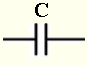# Capacitor Impedance Calculator

The impedance $Z_C$ of a capacitor of capacitance $C$, in complex form, is given by$Z_C = -j \; X_C$
where $j$ is the imaginary unit and $X_C = \dfrac{1}{ \omega C }$ is the capacitive reactance in Ohms $( \Omega )$
In , $Z_C$ is writtean as
$Z_C = X_C \; \angle \; - 90^{\circ}$ or $Z_C = X_C \; \angle \; - \dfrac{\pi}{2}$
$\omega = 2 \pi f$ is the angular frequency in radians per second (rad/s) and $f$ is the frequency in Hertz (Hz).
This calculator calculates angular frequency $\omega$, the capacitive reactance $X_C$ and the impedance $Z_C$ in complex standard and polar forms.

## Use of the calculator

Enter the capacitance $C$ and the frequency $f$ and press "Calculate".

 Capacitance C = 100 pF nF μF mF F Frequency f = 1.5 GHz MHz kH Hz mHz Number of Decimal Places = 5

## Results

in complex form
in polar form with phase in degrees
in polar form with phase in radians